The Fundamentals of Magnetism in Electronics

Key Terms

o         Magnetic force

o         Magnetism

o         Magnetic field

o         Induction

Objectives

o         Relate magnetism to electric current

o         Show how the magnetic field affects wire loops

No discussion of electric circuits would be complete without an introduction to magnetism, a phenomenon that underlies a variety of circuit devices such as inductors and transformers, as well as other devices you've probably encountered in daily life.

Elements of Magnetism

As a child, you may have played with magnets, and even as an adult, you probably use them every now and then (for instance, when attaching a note to your refrigerator). Magnets exert a force on each other and on certain metals (iron and certain types of steel, for instance): this force is called the magnetic force. What you may not know, however, is that magnetism has its source in electric charge.

Interested in learning more? Why not take an online Electronics course?

Two stationary charges exert an electric force on each other, but only when one charge is in motion relative to the other (i.e., when a current exists) is there a magnetic force. We'll not go into too much depth regarding the particulars of this force; truly understanding its relationship to charge is a very complicated task, both conceptually and mathematically.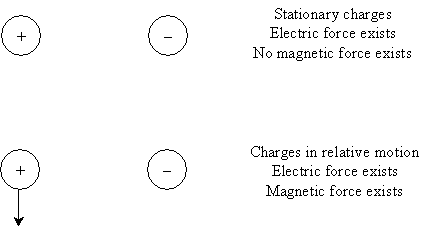Let's consider one practical example to illustrate how magnetism works in the context of a circuit. (Here, we'll be talking about the magnetic field, which is not quite the same as the magnetic force. For our purposes, however, we'll treat them as effectively the same.) Imagine a length of wire with a current flowing along it. The movement of positive charge creates a magnetic field that "circles" the wire and is strong close to the wire but weak far from the wire. The diagram below shows two views of the wire, current, and magnetic field.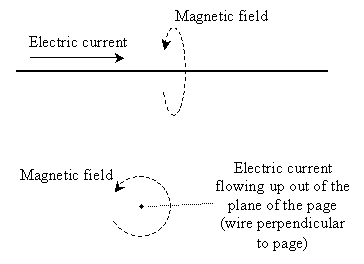The magnetic field "circles" the wire, as the diagram illustrates. This effect can also be seen in a wire "loop," where the magnetic field forms a "doughnut" shape.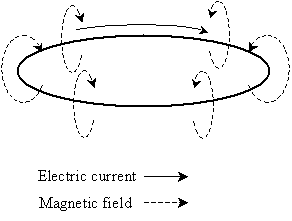Practical Effects of Magnetism

You probably know that a compass works by using the Earth's magnetic field to indicate direction. If you have a compass, a battery and a piece of wire, you can see for yourself how current creates a magnetic force. Connect a wire directly across the two terminals of the battery (thereby creating a large current through the wire) and hold it near the compass. You should see the compass needle move. When you disconnect the wire, the needle should return to its original orientation. (But don't leave the wire connected to the battery terminals too long--you'll quickly discharge the battery!) What you've created in this case with the battery and the wire is a simple electromagnet.

Let's return to the loop of wire discussed above. Alone, no current exists on the wire because there's no power supply creating a voltage.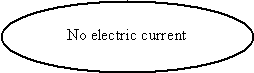We noted that if a current flows around the wire, a magnetic field is the result. But what if we expose the wire to a magnetic field? As it turns out, a constant magnetic field has no effect, but a changing magnetic field (for instance, it gets stronger or weaker) does have an effect: it creates a voltage around the wire, resulting in a current. The amount of current is related to the changing magnetic field through the wire loop. Thus, if we move a wire loop toward a magnet (resulting in an increasing magnetic field through the loop), or vice versa, a current is generated.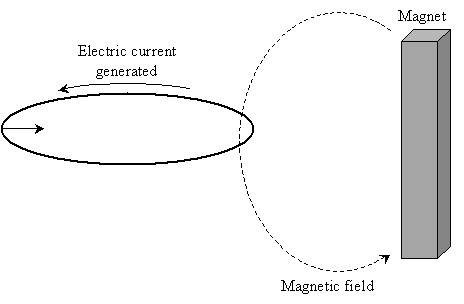The details of this concept are, again, complicated, but this phenomenon is how electricity is generated (a "wire loop" is moved in a magnetic field, creating a current that supplies homes, businesses, and so on). This creation of charge as a result of a changing magnetic field through a wire loop is called induction (a current is "induced" in the wire).

A Final Note on Induction

To conclude this ever so brief discussion of magnetism, consider what happens when we pass a current through a wire loop. A slightly more realistic scenario is shown below.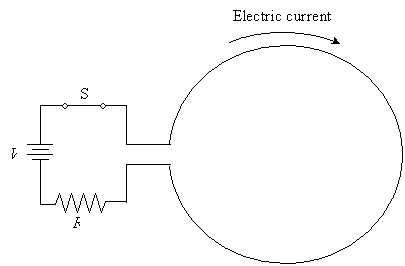Remember that we said an electric current creates a magnetic field, but when switch S is first closed, the current begins to flow, creating a magnetic field through the loop. But a changing magnetic field (increasing, in this case) creates a current--which could be troublesome if the current induced by the magnetic field is in the same direction as the current created by the power supply, as this would create a "chain reaction" leading to infinite current through the loop! As it turns out, the current induced by the changing magnetic field in this case is opposite that generated by the voltage supply. This induced current will "cancel" part of the generated (by the power supply) current until the current through the circuit reaches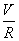(remember Ohm's law).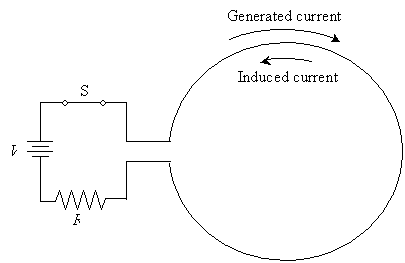Thus, when switch S is closed, the actual current through the loop increases over time torather than becomingimmediately.

Practice Problem: Consider the simple resistor circuit below. An electronics technician then places a wire loop next to this other circuit. What will happen to the wire loop when the technician moves it toward the circuit? Explain.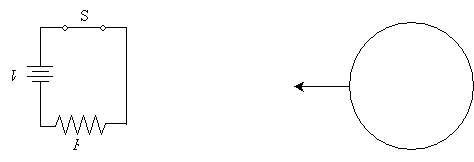Solution: Consider first the resistor circuit. Because S is closed, a current flows through the circuit. But recall that current creates a magnetic field; effectively, it is an electromagnet. As a result, as the technician moves the wire loop toward the circuit, the magnetic field through the loop changes (it increases as the loop gets closer to the circuit), thereby creating a current in the loop. Although we can't quantify the magnitude of the current or ascertain the direction at this point, we do know that a current is induced. This situation actually illustrates a challenge circuit designers face: the magnetic field from one circuit can actually "link" that circuit to another nearby circuit, effectively exchanging power! Although this linkage is negligible in many cases, it becomes particularly problematic in certain applications.